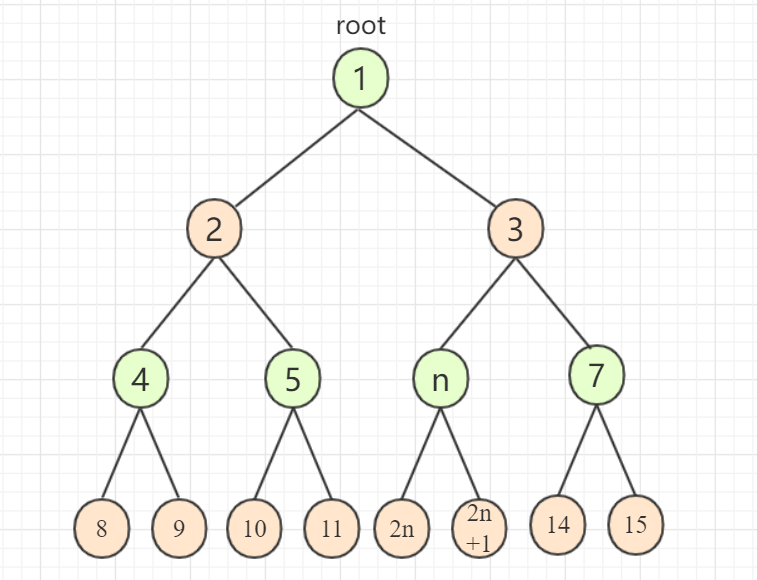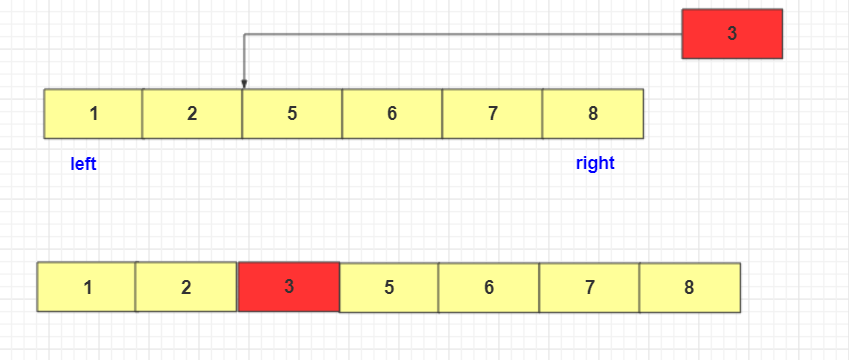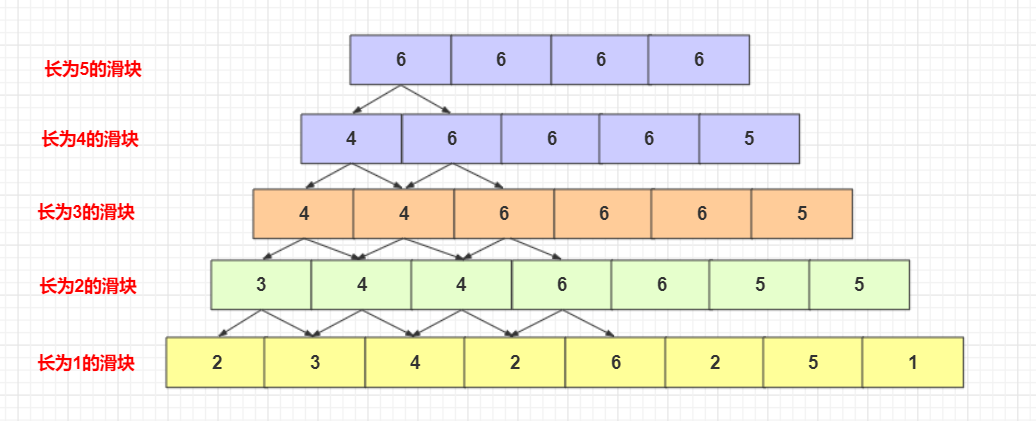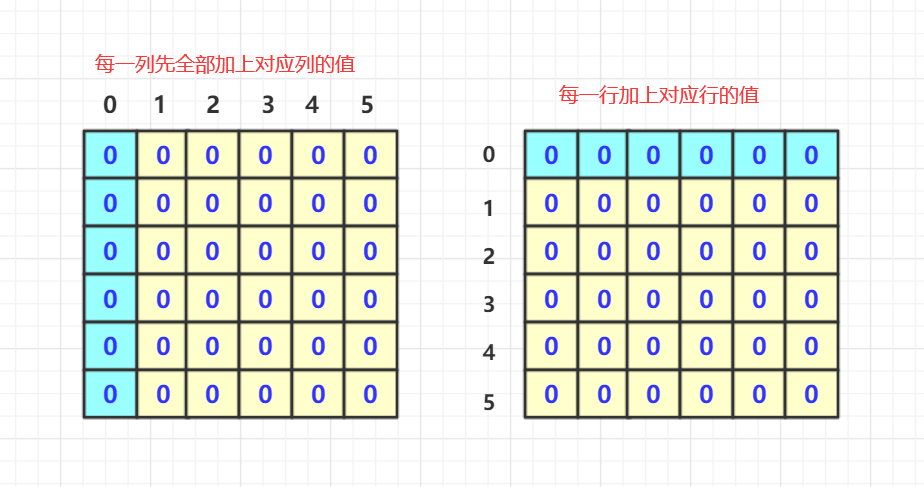# 剑指offer(60-67题)详解

### 文章目录

欢迎关注个人数据结构专栏
微信公众号：bigsai
剑指offer(1-10题)详解
剑指offer(11-25题)详解
剑指offer(26-33题)详解
剑指offer(34-40题)详解
剑指offer(41-50题)详解
剑指offer(51-59题)详解
剑指offer(60-67题)详解
声明：大部分题基本未参考题解，基本为个人想法，如果由效率太低的或者错误还请指正！到这里，剑指offer就搞完了2020-01-192020-02-29(大年初五)和100人一起，第一次组织吧，特记下！欢迎关注公众号：bigsai下次再一起玩！

## 60 把二叉树打印成多行

题目描述

从上到下按层打印二叉树，同一层结点从左至右输出。每一层输出一行。

思路
这题和上面59思路差不多，甚至更容易一些，对于层序遍历。需要分层，所以用2个队列进行区别一下层就可以了。

实现代码为：

import java.util.ArrayDeque;
import java.util.ArrayList;
import java.util.Queue;

/*
public class TreeNode {
int val = 0;
TreeNode left = null;
TreeNode right = null;

public TreeNode(int val) {
this.val = val;

}

}
*/
public class Solution {
ArrayList<ArrayList<Integer> > Print(TreeNode pRoot) {
ArrayList<ArrayList<Integer>>list=new ArrayList<ArrayList<Integer>>();
if(pRoot==null)return list;
Queue<TreeNode>q1=new ArrayDeque<TreeNode>();
Queue<TreeNode>q2=new ArrayDeque<TreeNode>();
while (!q1.isEmpty()||!q2.isEmpty()) {
ArrayList<Integer>list2=new ArrayList<Integer>();
while (!q1.isEmpty()) {
TreeNode team=q1.poll();
}
}
return list;
}
}


## 61 序列化二叉树

题目描述

请实现两个函数，分别用来序列化和反序列化二叉树

二叉树的序列化是指：把一棵二叉树按照某种遍历方式的结果以某种格式保存为字符串，从而使得内存中建立起来的二叉树可以持久保存。序列化可以基于先序、中序、后序、层序的二叉树遍历方式来进行修改，序列化的结果是一个字符串，序列化时通过 某种符号表示空节点（#），以 ！ 表示一个结点值的结束（value!）。

二叉树的反序列化是指：根据某种遍历顺序得到的序列化字符串结果str，重构二叉树。

思路
对于序列化的概念，大家肯定会想起面向对象啦，json之类的开发相关，序列化反序列化就是能够将一种结构变成字符存储，而反序列化就是能够根据制定的某种规则构造出原结构相同的对象

那么这题个人有两个想法吧，但是实现只实现了一个。

思路一
二叉树是可以用数组存储的，虽然用数组遇到不规则的二叉树空间利用效率会很低，但是是可以存的。对于第n个节点。它的两个儿子是2n2n+1(对应数组的物理地址)。

• 所以实现上序列化你建立一个TreeNode数组，然后从根节点向后遍历，如果有儿子放到对应位置。(当然这个实现上有点盲目性，不太好)。返回字符串如果有值返回对应值没值的话可以用个特殊字串占位置。
• 而反序列化你只需要把字符串先放到对应数组中，然后建立TreeNode数组与上面的遍历关联，如果有值那么就在这个位置建立TreeNode节点。再次遍历的时候如果第n个节点的left指向2n，right指向2n+1位置.最终返回第1个位置的节点即可！反序列化完成。思路二
我们知道：一个中序带着前序或者后序可以确定一个二叉树！好了，我们就用前序可中序完成。前面讲过二叉树的几种遍历。在非递归遍历的前序和中序可以共用一个过程！详细可以参考数据结构专栏哈！

• 序列化：给你个根节点，非递归前序和中序序列可以搞出来吧？但是记住字符串要有个东西分割，不能直接加。比如19 8中间必须有个东西分隔开来，这个要注意。最后直接将两个字符相加返回即可。
• 反序列化： 给你带有前序和中序的字符串。分割出前序和中序序列。前面第四题是构建二叉树就是用前序和中序构建二叉树的，就是递归的妙用，至于还原具体的思路还请还对应剑指offer的题解

实现代码为：

import java.util.Stack;
/*
public class TreeNode {
int val = 0;
TreeNode left = null;
TreeNode right = null;

public TreeNode(int val) {
this.val = val;
}
}
*/
public class Solution {
static  String Serialize(TreeNode root) {
if(root==null)return "";
String qian="";
String zhong="";

Stack<TreeNode>stack=new Stack<TreeNode>();

while (!stack.isEmpty()||root!=null) {//前序的
if(root!=null)
{
qian+=" "+root.val;
stack.push(root);
root=root.left;
}
else {
root=stack.pop();
zhong+=" "+root.val;
root=root.right;
}
}
return qian.substring(1)+zhong;

}
static TreeNode Deserialize(String str) {
if(str.equals(""))return null;
String value[]=str.split(" ");
int len=value.length;
int qian[]=new int [len/2];
int hou[]=new int [len/2];
for(int i=0;i<len/2;i++)
{
qian[i]=Integer.parseInt(value[i]);
}
for(int i=0;i<len/2;i++)
{
hou[i]=Integer.parseInt(value[i+len/2]);
}
return creat(qian,hou,0,len/2-1,0,len/2-1);
}
private static TreeNode creat(int[] pre, int[] in, int preleft, int preright, int inleft, int inright) {
if(preleft>preright||inleft>inright)return null;
TreeNode node=new TreeNode(pre[preleft]);
int mid=0;
for(int i=inleft;i<=inright;i++)
{
if(pre[preleft]==in[i])
{
mid=i;
}
}
node.left=creat(pre, in, preleft+1, preleft+(mid-inleft), inleft, mid-1);
node.right=creat(pre, in, preleft+(mid-inleft)+1, preright, mid+1, inright);
return node;
}
}


参考评论区：
有个递归方法挺好的，值得参考：链接：https://www.nowcoder.com/questionTerminal/cf7e25aa97c04cc1a68c8f040e71fb84?answerType=1&f=discussion
来源：牛客网

public class SerializeTree {

int index = -1;
/**
* 分别遍历左节点和右节点，空使用#代替，节点之间，隔开
*
* @param root
* @return
*/
public String Serialize(TreeNode root) {
if (root == null) {
return "#";
} else {
return root.val + "," + Serialize(root.left) + "," + Serialize(root.right);
}
}
/**
* 使用index来设置树节点的val值，递归遍历左节点和右节点，如果值是#则表示是空节点，直接返回
*
* @param str
* @return
*/
TreeNode Deserialize(String str) {
String[] s = str.split(",");//将序列化之后的序列用，分隔符转化为数组
index++;//索引每次加一
int len = s.length;
if (index > len) {
return null;
}
TreeNode treeNode = null;
if (!s[index].equals("#")) {//不是叶子节点 继续走 是叶子节点出递归
treeNode = new TreeNode(Integer.parseInt(s[index]));
treeNode.left = Deserialize(str);
treeNode.right = Deserialize(str);
}
return treeNode;
}

public static void main(String[] args) {
TreeNode treeNode1 = new TreeNode(1);
TreeNode treeNode2 = new TreeNode(2);
TreeNode treeNode3 = new TreeNode(3);
TreeNode treeNode4 = new TreeNode(4);
TreeNode treeNode5 = new TreeNode(5);
TreeNode treeNode6 = new TreeNode(6);

treeNode1.left = treeNode2;
treeNode1.right = treeNode3;

treeNode2.left = treeNode4;
treeNode3.left = treeNode5;
treeNode3.right = treeNode6;

SerializeTree serializeTree = new SerializeTree();

String str = serializeTree.Serialize(treeNode1);
TreeNode treeNode = serializeTree.Deserialize(str);
}
}


## 62 二叉搜索树第K个节点

题目描述

给定一棵二叉搜索树，请找出其中的第k小的结点。例如， （5，3，7，2，4，6，8） 中，按结点数值大小顺序第三小结点的值为4。

思路
首先，二叉搜索树是有序的！二叉搜索树的中序遍历是有序序列！(基础知识和常识了)。所以你主要非递归中序把节点释放到第K个就可以返回结束了。(也可使用递归版本)

实现代码为：

import java.util.Stack;
/*
public class TreeNode {
int val = 0;
TreeNode left = null;
TreeNode right = null;

public TreeNode(int val) {
this.val = val;
}
}
*/
public class Solution {
TreeNode KthNode(TreeNode pRoot, int k)
{
if(k==0)return null;
Stack<TreeNode>stack=new Stack<TreeNode>();
while (!stack.isEmpty()||pRoot!=null) {
if(pRoot!=null)
{
stack.push(pRoot);
pRoot=pRoot.left;
}
else {
pRoot=stack.pop();
k--;
if(k==0)return pRoot;
pRoot=pRoot.right;
}
}
if(k>0)return null;
return pRoot;
}
}


## 63 数据流中的中位数

题目描述

如何得到一个数据流中的中位数？如果从数据流中读出奇数个数值，那么中位数就是所有数值排序之后位于中间的数值。如果从数据流中读出偶数个数值，那么中位数就是所有数值排序之后中间两个数的平均值。我们使用Insert()方法读取数据流，使用GetMedian()方法获取当前读取数据的中位数。

思路
构造类型的题，中位数，也就是要我们维护一个有序数列取中间数。其实实现的方法也比较多吧。比如你可以用Arraylist。每次加入快速排序。也可以用优先队列进行堆排序维护。但是快排对已经有序序列效率不高，队列取数是比较麻烦的。

当然，注意这个序列是你一手构造的，从开始为0，并且每次加入节点的序列都是有序的。其实插入排序更好，这个步骤就相当于插入排序的最后一部一样。把最后一个找到合适位置插入就可以了。而序列是有序的，二分法+直接插入是一种很不错的解法！实现代码为：

import java.util.ArrayList;
import java.util.List;
public class Solution {
List<Integer> list = new ArrayList<Integer>();
public void Insert(Integer num) {
int l=0,r=list.size()-1;
int mid=(l+r)/2;
while (l<r) {
if(list.get(mid)<num)
{
l=mid+1;
mid=(l+r)/2;
}
else if (list.get(mid)>num) {
r=mid;
mid=(l+r)/2;
}
else {
l=r=mid;
}
}
}
public Double GetMedian() {
if(list.size()%2==1)
{
return (double)list.get(list.size()/2);
}
else {
return ((double) (list.get((list.size()-1)/2)+list.get(list.size()/2))/2);
}
}
}


## 64 滑动窗口的最大值

题目描述

给定一个数组和滑动窗口的大小，找出所有滑动窗口里数值的最大值。例如，如果输入数组{2,3,4,2,6,2,5,1}及滑动窗口的大小3，那么一共存在6个滑动窗口，他们的最大值分别为{4,4,6,6,6,5}； 针对数组{2,3,4,2,6,2,5,1}的滑动窗口有以下6个： {[2,3,4],2,6,2,5,1}， {2,[3,4,2],6,2,5,1}， {2,3,[4,2,6],2,5,1}， {2,3,4,[2,6,2],5,1}， {2,3,4,2,[6,2,5],1}， {2,3,4,2,6,[2,5,1]}。

思路
这题有技巧的，可以直接查找但是可能混乱一点。这题有兴趣的可以了解线段树，感觉有些思想有点像。虽然蛮干和我的题解在效率差别不大，但是这个思想感觉可以体会一下。

• (1)如果区间为1，那么最大的就是每个数。
• (2)如果区间为2，就是相邻的2个进行比较滑动取最大。
• (3)如果区间为3，就是相邻的3个进行比较！还是相邻的(2)中的相邻两个最大两个滑动。
• (4)如果区间为4，就是相邻的4个进行比较！还是相邻的(3)中的相邻两个最大两个滑动。
• -----------

比如序列2 3 4 2 6 2 5 1

• 区间为1：2 3 4 2 6 2 5 1
• 区间为2：(23)3 (34)4 (42)4 (26)6 (62)6 (25)5 (51)5 7个窗口
• 区间为3：(234)3 (342)4 (426)6 (262)6 (625)6 (251)5 6个滑动。也等价于 (2334)3 (3442)4 (4226)6 (2662)6 (6225)6 (2551)5（区间为2的相互比较）。

当然在空间利用方面，其实不需要开辟新的数组，直接重复利用一个数组就行了，算完一轮进行下一轮，每次长度你记住少一就行了。实现代码为：

import java.util.ArrayList;
public class Solution {
public static ArrayList<Integer> maxInWindows(int [] num, int size)
{
ArrayList<Integer>list=new ArrayList<Integer>();
if(size==0)return list;
int len=num.length;
while (size-->1) {
for(int i=0;i<len-1;i++)
{
num[i]=Math.max(num[i], num[i+1]);
}
len--;
}
System.out.println(len);

for(int i=0;i<len;i++)
{
}
return list;
}

}


参考评论区：
评论区的双队列方法：

链接：https://www.nowcoder.com/questionTerminal/1624bc35a45c42c0bc17d17fa0cba788?f=discussion
来源：牛客网

/**
* 双队列方法
* 滑动窗口的最大值总是保存在队列首部，队列里面的数据总是从大到小排列。
*
* [@param num
* @param size
* @return](/profile/547241) */
public ArrayList maxInWindows(int[] num, int size) {
ArrayList res = new ArrayList();
if (num == null || num.length == 0 || size == 0 || size > num.length) {
return res;
}
for (int i = 0; i < num.length; i++) {
if (!deque.isEmpty()) {
// 如果队列头元素不在滑动窗口中了，就删除头元素
if (i >= deque.peek() + size) {
deque.pop();
}
// 如果当前数字大于队列尾，则删除队列尾，直到当前数字小于等于队列尾，或者队列空
while (!deque.isEmpty() && num[i] >= num[deque.getLast()]) {
deque.removeLast();
}
}
deque.offer(i); // 入队列
// 滑动窗口经过一个滑动窗口的大小，就获取当前的最大值，也就是队列的头元素
if (i + 1 >= size) {
}
}
return res;
}


## 65 矩阵中的路径

题目描述

请设计一个函数，用来判断在一个矩阵中是否存在一条包含某字符串所有字符的路径。路径可以从矩阵中的任意一个格子开始，每一步可以在矩阵中向左，向右，向上，向下移动一个格子。如果一条路径经过了矩阵中的某一个格子，则该路径不能再进入该格子。 例如 a b c e s f c s a d e e 矩阵中包含一条字符串"bcced"的路径，但是矩阵中不包含"abcb"路径，因为字符串的第一个字符b占据了矩阵中的第一行第二个格子之后，路径不能再次进入该格子。

思路
基础迷宫类dfs搜素题。它给的是一维数组，我们要根据位置对应转换成二维的数组储存地图。为了方便我们进行搜素。另外要建立X[]Y[]表示上下左右四个移动方向。

而对于这类题我们的一般做法是：

• 二维的迷宫，用个boolean[][]数组判断对应位置在当前是否走过。
• 本题要求找到这么一串符合的，我们可以遍历首字母相同的，从首字母相同的进行深度优先搜索，按照方向符合就往下搜索，到整个串路径存在即可。
• 本题的搜索是属于试探回溯的。所以在dfs搜索走完一个位置如果满足条件，那么向四个满足条件(不越界没走过等)方向进行搜索，同时标记此位置在这条路径不能再次使用。而这条搜索完需要将boolean数组还原。至于dfs和回溯如果没基础的可以百度学习一下。

另外就是注意下特殊值，或者不满足的要判断排除。

实现代码为：

public class Solution {
boolean judge=false;//判断是否存在
int x[]= {-1,0,1,0};
int y[]= {0,1,0,-1};

public  boolean hasPath(char[] matrix, int rows, int cols, char[] str)
{
if(str.length==0||matrix.length==0)return false;
char map[][]=new char[rows][cols];
boolean jud[][]=new boolean[rows][cols];
for(int i=0;i<matrix.length;i++)//转到二维数组
{
map[i/cols][i%cols]=matrix[i];
}
for(int i=0;i<rows;i++)
{
for(int j=0;j<cols;j++)
{
if(map[i][j]==str)//第一个相同
{
dfs(map,jud,i,j,0,str);
if(judge)return true;
}
}
}
return false;
}
private  void dfs(char[][] map, boolean[][] jud, int m, int n, int index, char[] str) {
if(index==str.length-1) {if(map[m][n]==str[index])judge=true;}//有成功的
else if(map[m][n]!=str[index]) {}//回溯停止
else {//map[n][n]==str[index]
jud[m][n]=true;
for(int i=0;i<4;i++)
{
if(m+x[i]>=0&&m+x[i]<map.length&&n+y[i]>=0&&n+y[i]<map.length&&map[m+x[i]][n+y[i]]==str[index+1])
{
if(!jud[m+x[i]][n+y[i]])
{
dfs(map, jud, m+x[i], n+y[i], index+1, str);
}
}
}
jud[m][n]=false;
}
}
}


## 66 机器人的运动范围

题目描述

地上有一个m行和n列的方格。一个机器人从坐标0,0的格子开始移动，每一次只能向左，右，上，下四个方向移动一格，但是不能进入行坐标和列坐标的数位之和大于k的格子。 例如，当k为18时，机器人能够进入方格（35,37），因为3+5+3+7 = 18。但是，它不能进入方格（35,38），因为3+5+3+8 = 19。请问该机器人能够达到多少个格子？

思路
这题也是简单搜索题，但是处理方式可以用bfs，也可以用dfs主要考察迷宫的通达性。所以你用dfs千万不要将Boolean判断数组复原，走过的地方只能走一次。至于这个规则我想很容易判断，求余10和除10下去就可以计算每个位置是否满足规则。

但是如果迷宫和K足够大的话，那么横纵坐标都这要这么计算n次，效率是不高的。你可以预处理的，每一行每一列先加上对应行和列。这样搜索时候不需要一个个计算直接比较就可以了。当然如果K小或者迷宫小可能会造成一些无用计算，这里了解下就可以了(并未采用)。实现代码为：

public class Solution {
int X[]= {-1,0,1,0};
int Y[]= {0,1,0,-1};
int num=0;
public int movingCount(int threshold, int rows, int cols)
{
boolean jud[][]=new boolean[rows][cols];
dfs(0,0,rows,cols,threshold,jud);
return num;
}
private void dfs(int x, int y, int rows, int cols, int threshold, boolean[][] jud) {
if(count>threshold) { }
else {
num++;
jud[x][y]=true;
for(int i=0;i<4;i++)
{
if(x+X[i]>=0&&x+X[i]<rows&&y+Y[i]>=0&&y+Y[i]<cols&&!jud[x+X[i]][y+Y[i]])//不越界
{
dfs(x+X[i], y+Y[i], rows, cols, threshold, jud);
}
}
}
}
private int nadd(int x, int y) {//计算个位数字之和
int num=0;
while (x>0) {
num+=x%10;x/=10;
}
while (y>0) {
num+=y%10;y/=10;
}
return num;
}

}


## 67 剪绳子

题目描述

给你一根长度为n的绳子，请把绳子剪成整数长的m段（m、n都是整数，n>1并且m>1），每段绳子的长度记为k,k,…,k[m]。请问kxkx…xk[m]可能的最大乘积是多少？例如，当绳子的长度是8时，我们把它剪成长度分别为2、3、3的三段，此时得到的最大乘积是18。
输入描述:
输入一个数n，意义见题面。（2 <= n <= 60）

输出描述:

输出答案。
示例1
输入
8
输出
18

思路
数据范围很小，还没看题解过得，盲猜贪心思路！谈谈灵感吧(不一定对)！

• 如果分成两份，两个相同的乘积应该最大
• 如果分成n份，n个相同乘积应该最大
• 分成几份？我猜对于一个数N，mm=n这个应该是最优等分。但是m只能取整数，所以一定在m取整的左右之间！好了，n<60这个范围太小。很好搞。也就分成几个区间而已.甚至2和3段分法能数出来是7.3*4=12,2*3*3=12.所以7到27肯定3段。27-60在3和4不确定，比较一下就行了。

知道分段n均分会求吧！这里有个小技巧可以参考代码。

 int a[]= {1,4,27,128};//1 2*2 3*3*3 4*4*4*4    2-7分两个  7-27分3   27-60分3或者4尝试


实现代码为：

public class Solution {
public int cutRope(int target) {
int a[]= {1,4,27,128};//1 2*2  3*3*3  4*4*4     2-7分两个     7-27分3    27-60分3或者4尝试
if(target<=7)
return (target/2)*(target-target/2);
else if (target<=27) {
return (target/3)*((target+1)/3)*((target+2)/3);//看看余数要分配给几个数
}
else {
return Math.max((target/3)*((target+1)/3)*((target+2)/3), (target/4)*((target+1)/4)*((target+2)/4)*((target+3)/4));
}
}
}


参考评论区
看了评论区才发现原来这题分析的正解是什么，前面的自己做法虽然A了但可能是错误，但是这个想法仍有部分参考价值吧

主流解法是贪心和dp吧。个人更推荐贪心感觉更容易理解。

链接：https://www.nowcoder.com/questionTerminal/57d85990ba5b440ab888fc72b0751bf8?f=discussion
来源：牛客网

//
// Created by yuanhao on 2019-9-3.
//

#include <iostream>
#include <cmath>

using namespace std;

/**
* 题目分析：
* 先举几个例子，可以看出规律来。
* 4 ： 2*2
* 5 ： 2*3
* 6 ： 3*3
* 7 ： 2*2*3 或者4*3
* 8 ： 2*3*3
* 9 ： 3*3*3
* 10：2*2*3*3 或者4*3*3
* 11：2*3*3*3
* 12：3*3*3*3
* 13：2*2*3*3*3 或者4*3*3*3
*
* 下面是分析：
* 首先判断k到k[m]可能有哪些数字，实际上只可能是2或者3。
* 当然也可能有4，但是4=2*2，我们就简单些不考虑了。
* 5<2*3,6<3*3,比6更大的数字我们就更不用考虑了，肯定要继续分。
* 其次看2和3的数量，2的数量肯定小于3个，为什么呢？因为2*2*2<3*3，那么题目就简单了。
* 直接用n除以3，根据得到的余数判断是一个2还是两个2还是没有2就行了。
* 由于题目规定m>1，所以2只能是1*1，3只能是2*1，这两个特殊情况直接返回就行了。
*
* 乘方运算的复杂度为：O(log n)，用动态规划来做会耗时比较多。
*/
long long n_max_3(long long n) {
if (n == 2) {
return 1;
}
if (n == 3) {
return 2;
}
long long x = n % 3;
long long y = n / 3;
if (x == 0) {
return pow(3, y);
} else if (x == 1) {
return 2 * 2 * (long long) pow(3, y - 1);
} else {
return 2 * (long long) pow(3, y);
}
}

//给你一根长度为n的绳子，请把绳子剪成m段（m、n都是整数，n>1并且m>1），每段绳子的长度记为k,k,...,k[m]。请问kxkx...xk[m]可能的最大乘积是多少？例如，当绳子的长度是8时，我们把它剪成长度分别为2、3、3的三段，此时得到的最大乘积是18。
//
//输入描述:
//输入一个数n，意义见题面。（2 <= n <= 100）
//
//
//输出描述:
//输出答案。
//示例1
//输入
//8
//输出
//18
int main() {
long long n = 0;
cin >> n;
cout << n_max_3(n) << endl;
return 0;
}

链接：https://www.nowcoder.com/questionTerminal/57d85990ba5b440ab888fc72b0751bf8?f=discussion
来源：牛客网

/**
* @param target
* @return
*
* 分类：最值型、划分型
*
* 考虑最后一步：
* 必然有一个点，把target分成两段，两段分别构成最小子问题。
* 两段的最大值的乘积，也是target所求的最大值。
* 设划分点为i,f[i]表示长度为i的绳子的乘积最大值。
*
* 转移方程：
* f[i] = MAX{f[j]*f[i-j]}|0<j<i
*
* 那么我们求的就是f[target]
*
* 初始值：
* f=1
* f=1
*
* 边界情况：
* 无
*
* 计算顺序:
* i从1到target
* j从1到i
*/
public int cutRope(int target) {
int[] f = new int[target+1];
//初始化
f = 0;
f = 1;
for (int i = 1; i <= target; i++) {
/**
* 处理不分割的情况，因为绳子不能不被分割
*/
if(i==target) {
f[i] = 1;
}else {
f[i] = i;
}
for (int j = 1; j < i; j++) {
f[i] = Math.max(f[i],f[j]*f[i-j]);
}
}
return f[target];
}



到这里，剑指offer就搞完了。2020-01-19到2020-02-29(大年初五)，特记！欢迎关注公众号：bigsai下次再一起玩！

还能输入1000个字符
©️2019 CSDN 皮肤主题: 代码科技 设计师: Amelia_0503扫一扫，手机浏览